Latest Banking jobs   »

# Quantitative Aptitude Quiz For IDBI AM/Executive 2022- 24th June

Directions (1-5): In each of these questions, two equations are given. You have to solve both the equations and give answer
(a) if x>y
(b) if x≥y
(c) if x = y or no relation can be established between x and y.
(d) if y>x
(e) if y≥x

Quantitative Quiz For IDBI AM/Executive 2022 24th June CHECK IN HINDI

Q1. (i) x² – 12x + 32 = 0
(ii) y² – 20y + 96 = 0

Q2. (i) 2x² – 3x – 20 = 0
(ii) 2y² + 11y + 15 = 0

Q3. (i) x² – x – 6 = 0
(ii) y² – 6y + 8 = 0

Q4. (i) x² + 14x – 32 = 0
(ii) y² – y – 12 = 0

Q5. (i) x² – 9x + 20 = 0
(ii) 2y² – 12y + 18 = 0

Directions (6-10): In each of these questions, two equations (I) and (II) are given. You have to solve both the equations and give answer
(a) if x>y
(b) if x≥y
(c) if x<y
(d) if x ≤y
(e) if x = y or no relation can be established between x and y.

Q6. I. √625x² –375=0
II. √2116 y –736=0

Q7. I. 3x² + 5x + 2 = 0
II. 12y² + 11y + 2 = 0

Q8. I. 16x + 11y = 154
II. 11x + 16y = 89

Q9. I. 3x² + 14x – 5 = 0
II. 3y² – 19y + 6 = 0

Q10. I. 7x² – 11x – 6 = 0
II. 7y² – 10y + 3 = 0

Directions (11-15): In the following questions, two equations numbered I and II are given. You have to solve both the equations and give answers among the following options.
(a) if x > y
(b) if x ≥ y
(c) if x < y
(d) if x ≤ y
(e) if x = y or the relationship can’t be established.

Q11. I. 4x² – 7x – 15 = 0
II. 9y² + 33y + 18 = 0

Q12. I. x² =169
II. y² =26y-169

Q13. I. x² – 234.24 – 161.14 = 133.62
II. y = ∛12167

Q14. I. 11x² + 19x – 6 = 0
II. 4y² + 12y = 16

Q15. I. 12x² – 7x = –1
II. 6y² – 7y + 2 = 0

Solutions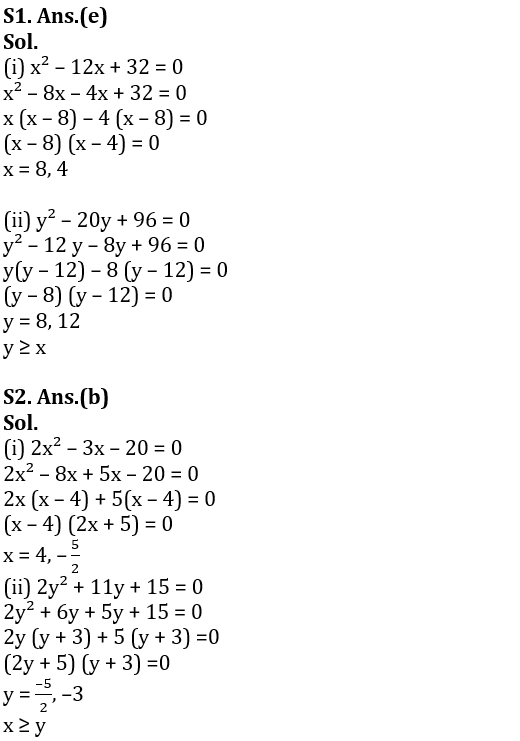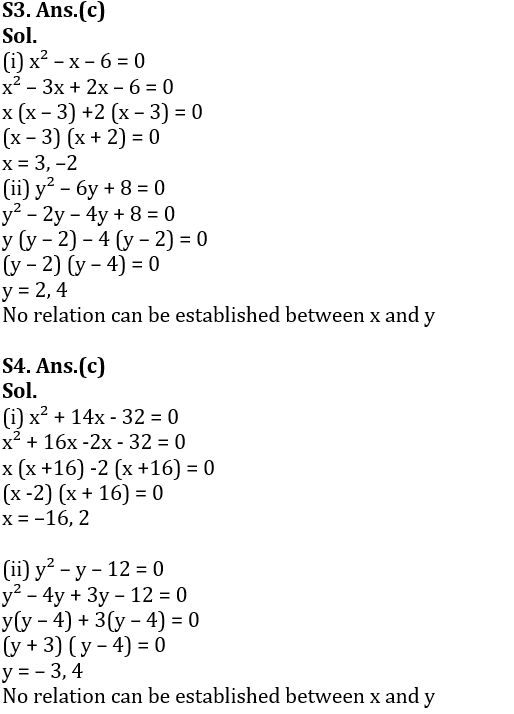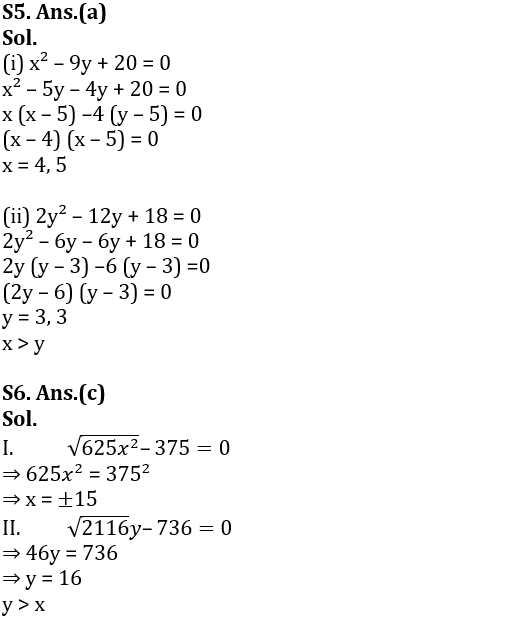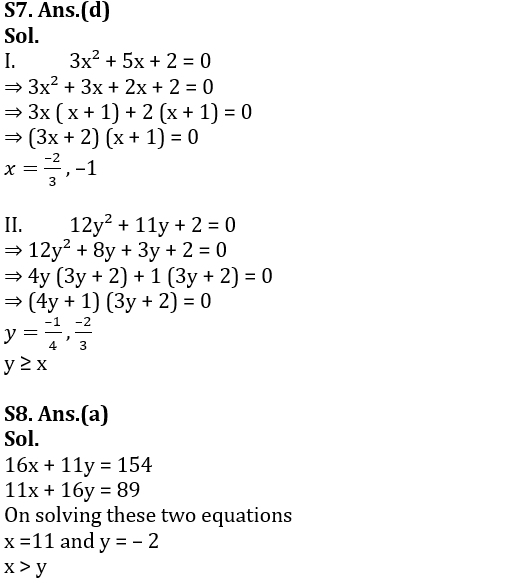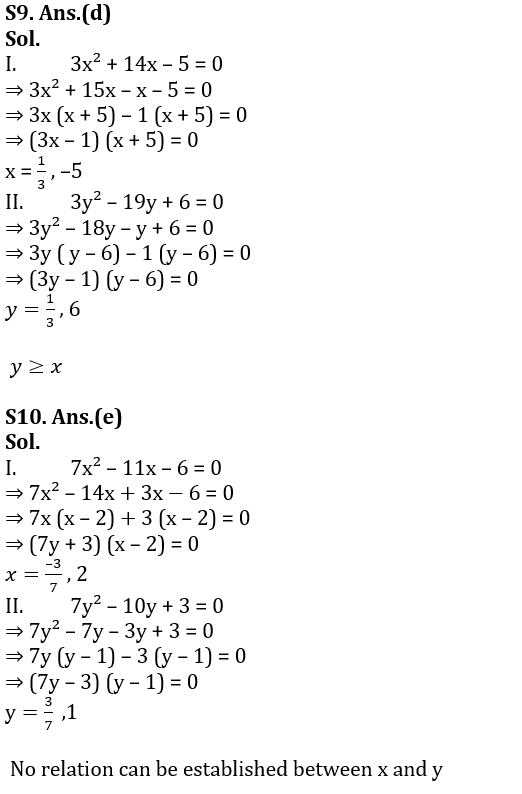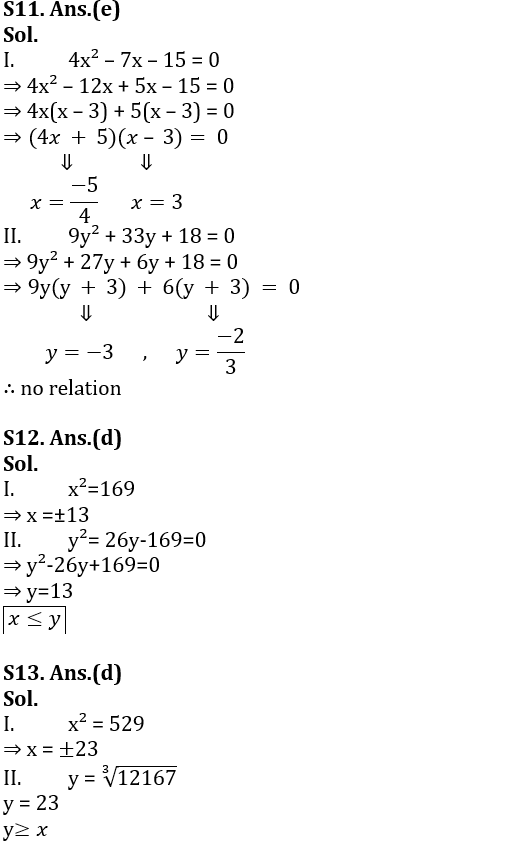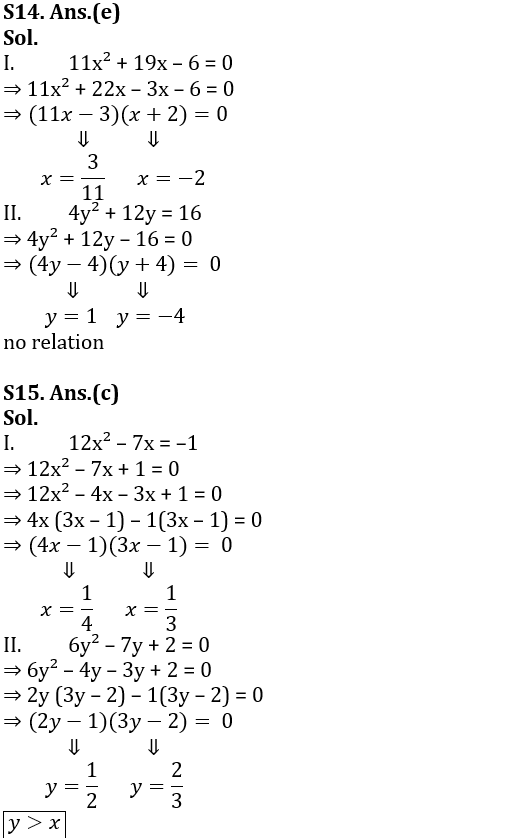#### Congratulations!